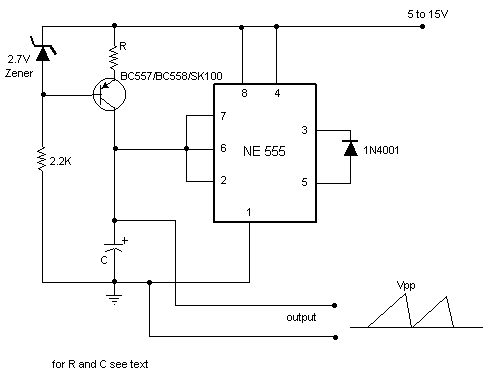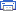# Sawtooth wave generator Electronic Schematics > Oscillators and timers > Sawtooth wave generatorSawtooth wave generators using opamp are very common. But the disadvantage is that it requires a bipolar power supply.

A sawtooth wave generator can be built using a simple 555 timer IC and a transistor as shown in the circuit diagram.

Самая актуальная информация granite countertop stores near me на сайте.

The working of the circuit can be explained as follows:
The part of the circuit consisting of the capacitor C, transistor,zener diode and the resistors form a constant current source to charge the capacitor. Initially assume the capacitor is fully discharged. The voltage across it is zero and hence the internal comparators inside the 555 connected to pin 2 causes the 555's output to go high and the internal transistor of 555 shorting the capacitor C to ground opens and the capacitor starts charging to the supply voltage. As it charges, when its voltage increases above 2/3rd the supply voltage, the 555's output goes low, and shorts the C to ground, thus discharging it. Again the 555's output goes high when the voltage across C decreases below 1/3rd supply. Hence the capacitor charges and discharges between 2/3rd and 1/3rd supply.

Note that the output is taken across the capacitor. The 1N4001 diode makes the voltage across the capacitor go to ground level (almost).

The frequency of the circuit is given by:

f = (Vcc-2.7)/(R*C*Vpp)

where:

Vcc= Supply voltage.
Vpp= Peak to peak voltage of the output required.

Choose proper R,C,Vpp and Vcc values to get the required 'f' value.

Title: Sawtooth wave generator
electronic circuit
Source: www.electronic-circuits-diagrams.com
Published on: 2005-02-01
Print version: